#Quality Digest

Featured Product
This Week in Quality Digest Live
Statistics Features
Donald J. Wheeler
What do predictable processes have in common with chaos?
William A. Levinson
People can draw the wrong conclusions due to survivor, survey, and bad news bias.
Donald J. Wheeler
Does your approach do what you need?
Paul Laughlin
Correlation vs. causality
Donald J. Wheeler
In spite of what everyone says to the contrary
Statistics News
New capability delivers deeper productivity insights to help manufacturers meet labor challenges
Day and a half workshop to learn, retain, and transfer GD&T knowledge across an organization
Elsmar Cove is a leading forum for quality and standards compliance
InfinityQS’ quality solutions have helped cold food and beverage manufacturers around the world optimize quality and safety
User friendly graphical user interface makes the R-based statistical engine easily accessible to anyone
Collect measurements, visual defect information, simple Go/No-Go situations from any online device
Good quality is adding an average of 11 percent to organizations’ revenue growth
Ability to subscribe with single-user minimum, floating license, and no long-term commitment
A guide for practitioners and managersBio

Statistics

## Data Snooping, Part 2

### What pitfalls lurk outside your database?

Published: Monday, September 10, 2018 - 12:03

In “Data Snooping Part 1” (Quality Digest, Aug. 6, 2018) we discovered the basis for the first caveat of data snooping. Here we discover three additional caveats of data snooping.

Last month we discovered:Here we will use the data set from Part One to illustrate three additional caveats. The response variable Y represents the weekly steam usage for a chemical plant. X1 represents the amount of fatty acid in storage. X2 represents the amount of glycerin produced. X3 is the weekly number of hours of operation for the plant. (Last month an additional variable was included in the data set, but here we leave it out to illustrate what its absence does to our analysis.) As before, we use the first eight weeks of production as our baseline.Figure 1:  Baseline: Four variables for eight weeks of production

In figure 2 we see that each of the three simple regressions has a p-value that is less than 0.05. Furthermore, each of these regression models can explain more than 80 percent of the variation in YFigure 2:  Three simple linear regressions

In Part One, using these baseline data, we found that regressions using two independent variables could not really do better than using either Y = f(X2) or Y = f(X3). Since Y represents the amount of steam used, and X2 represents the amount of glycerin produced each week, let us use Y = f(X2) for our predictions. The specific equation for this model is: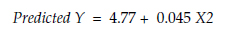Figure 3 shows this regression equation and the scatterplot for the baseline period. As expected, this regression model does a reasonable job of fitting these data.Figure 3:  Regression of Y upon X2 for baseline period

The data for weeks 9 through 25 are shown in figure 4.Figure 4:  Data for weeks 9 through 25

When we pair up the X2 and Y values from figure 4 we get the 17 points shown in red in figure 5. Clearly our regression equation from the baseline period does not fit these data. Perhaps it needs tweaking.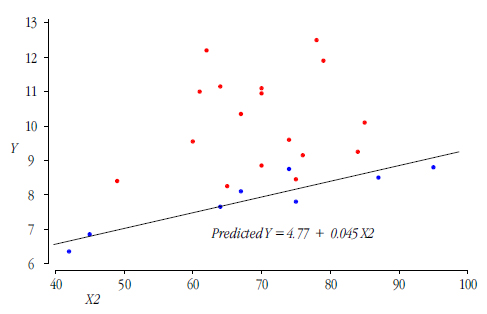Figure 5:  Baseline regression Y = f(X2) with additional data plotted

When we use all 25 weeks of data we find the simple regressions shown in figure 6. With a p-value of 0.138 the “relationship” between Y and X2 is found to be statistically indistinguishable from a horizontal line.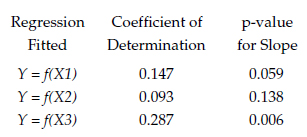Figure 6:  Three simple linear regressions for all 25 weeks

So while we found a strong relationship between Y and X2 in the baseline period, this relationship evaporates over time. This serves to illustrate the second caveat:This caveat effectively pulls the rug out from under using the results of data snooping for making predictions. Even if we split our database into separate portions, and use one portion to “confirm” what we found in the other portion, all of our data will still be historical, and any relationships we confirm will still only describe the past. Since all of the questions of interest will pertain to the future, our models of the past may not be useful for making predictions.

### What if we use what we find?

In figure 6 only Y = f(X3) shows a detectably non-zero slope. This simple regression explains 28.7 percent of the variation in Y. Can we do better with a bivariate regression? Figure 7 shows the results for adding a second variable to the model Y = f(X3) (using all 25 records).

The bivariate regression model Y = f(X3, X1) explains 28.8 percent of the variation in the response variable Y, but the conditional p-value for using X1 in addition to X3 is 0.90, which means that this bivariate regression model is not detectably better than Y = f(X3).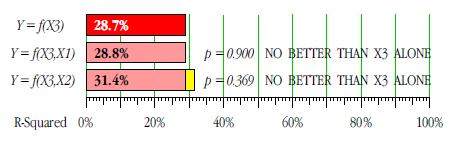Figure 7:  Adding variables to Y = f(X3)

The bivariate regression model Y = f(X3, X2) explains 31.4 percent of the variation in the response variable Y, but the conditional p-value for using X2 in addition to X3 is 0.369, which means that this bivariate regression model is not detectably better than Y = f(X3).

Since neither Y = f(X3, X1)  nor Y = f(X3, X2) does any better than Y = f(X3) we might decide to use Y = f(X3). This regression equation is:Figure 8:  Regression of Y upon X3

When we consider the scatterplot in figure 8 we immediately see that the regression of Y upon X3 is dominated by the two extreme points on the left. Remove these two points representing short production runs and the relationship between X3 and Y will vanish. While short production runs clearly result in less steam usage, there is no useful relationship here apart from these two abnormal weeks. This is a common problem of fitting regression models in any situation. Outliers can corrupt your model, and the only antidote is taking the time to look at the scatterplots. This illustrates the third caveat.At this point our data snooping has led us down two dead ends. First the strong relationship that we found in the baseline data vanished as additional data became available. Then as we analyzed our combined data set we found nothing but a relationship that was dependent upon outliers. In other words, while our data snooping resulted in a handful of regression equations, none of them had any hope of ever being useful in practice.

So even though the data will surrender if you torture them long enough, there is no guarantee that you will find anything useful when you go data snooping in messy data sets.

### What happens when we add X4?

In Part One we had an additional variable in our data set that represented the weekly average ambient atmospheric temperature. There, using all the data, we found that the simple regression of steam usage upon temperature, Y = f(X4) explained 71 percent of the variation in steam usage. When we added the amount of product produced, X2, we got a bivariate regression that explained 85 percent of the variation in Y. This bivariate regression equation is: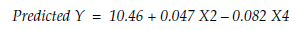A plot of these predicted values vs. the observed Y values is shown in figure 9.Figure 9:  Predicted Y vs. observed Y for Y = f(X2, X4)

So while there is a strong relationship between the steam usage and the temperature, and while the temperature combines with the amount of product produced to give a good approximation to the observed steam usages, these relationships cannot be discovered when the temperature data are not included in the data set. And this illustrates the fourth caveat:### The caveats of data snooping

Parts One and Two have illustrated four caveats for data snooping. The first and fourth caveats pertain to what we may miss. The second and third have to do with what we may find in error. These caveats are sufficiently well known to have names.With an existing data set, your variables will only take on those levels that have occurred in the past. When these past levels are restricted in some way you may well overlook some important relationships while modeling relationships of lesser import.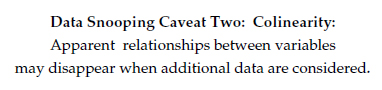Some apparent relationships may be nothing more than serendipity, but there is more to this caveat than a warning about accidental alignments. Messy data sets will generally have what mathematicians call a non-orthogonal data structure. These structures can cause the variation attributable to one variable to appear to be due to another variable. When variables exhibit colinearity (aka confounding), or when we have an accidental alignment between variables, the apparent relationships we have found may morph, shift, and change as additional data are added.This is a classic problem where one or two extreme points may create apparent relationships where none really exists. Many different types of regression routines have been developed in attempts to make regression more robust to outliers. But the simple scatterplot still remains the best way to avoid using a model that is highly dependent upon outliers.With all of our mathematical theory, and all of our software, we still do not know how to incorporate unknown independent variables into our regression models.

### Summary

Existing data sets are always messy. As we include more independent variables in our data set, and as the number of levels for each variable increases, the number of possible combinations of variable levels will increase geometrically. As a result, as a database includes more variables it will typically have an increasing number of missing combinations of variable levels. These missing combinations will create non-orthogonal data structures, which will challenge our abilities to extract information about relationships from the data. So, with all these caveats, how can we ever analyze existing data sets?

First, we should not attempt to use data snooping unless we have some idea that needs to be examined in the light of the existing data. If we do not know what we are looking for, we are going to have a hard time finding anything in a messy data set.

Second, we cannot ever establish or prove that a given relationship exists using an existing data set. We can only identify possible relationships to be considered for experimental studies or to be validated by additional data sets.

As with everything in science, when different lines of evidence converge on a given result, that result gains credibility. This applies to the results of data snooping as well as the results of experimental studies.

Nevertheless, the caveats listed here mean that no single bit of data snooping can ever be conclusive. We simply cannot use data snooping to establish or prove that a specific relationship exists. And this shortcoming of data snooping is why my fellow statisticians do not like “observational studies.”

Yet, there is a way to utilize observational studies in spite of these caveats. This approach will be the topic of Part Three.

### Discuss